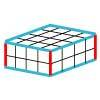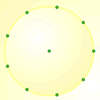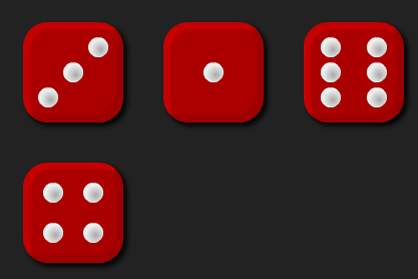#### You may also like### Round and Round the Circle

What happens if you join every second point on this circle? How about every third point? Try with different steps and see if you can predict what will happen.### Making Cuboids

Let's say you can only use two different lengths - 2 units and 4 units. Using just these 2 lengths as the edges how many different cuboids can you make?How many DIFFERENT quadrilaterals can be made by joining the dots on the 8-point circle?

# Round the Four Dice

##### Age 7 to 11Challenge LevelThere are four dice, each of them with faces labelled from 1 to 6.
When the dice are rolled they can be combined in twenty four different ways to make a four-digit number.
For example, if I roll a 1, 2, 3 and 4 I can combine them to make:
1234, 1243, 1324, 1342, 1423, 1432, 2134, 2143, 2314, 2341, 2413, 2431, 3124, 3142, 3214, 3241, 3412, 3421, 4123, 4132, 4213, 4231, 4312 or 4321.

Now pick four different four-digit numbers from the list and round each of them to the nearest multiple of 1000. For example, 1324 rounds to 1000, 2314 rounds to 2000, 4312 rounds to 4000 and 4123 rounds to 4000.
Do the four four-digit numbers you choose ever all round to the same multiple of 1000?
Do the four four-digit numbers you choose ever round to unique multiples of 1000?